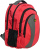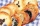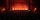# Fraction calculator

The calculator performs basic and advanced operations with fractions, expressions with fractions combined with integers, decimals, and mixed numbers. It also shows detailed step-by-step information about the fraction calculation procedure. Solve problems with two, three, or more fractions and numbers in one expression.

## Result:

### 2/3 * 3/4 = 1/2 = 0.5

Spelled result in words is one half.

### How do you solve fractions step by step?

1. Multiple: 2/3 * 3/4 = 2 · 3/3 · 4 = 6/12 = 1 · 6/2 · 6 = 1/2
Multiply both numerators and denominators. Result fraction keep to lowest possible denominator GCD(6, 12) = 6. In the following intermediate step, cancel by a common factor of 6 gives 1/2.
In other words - two thirds multiplied by three quarters = one half.

#### Rules for expressions with fractions:

Fractions - simply use a forward slash between the numerator and denominator, i.e., for five-hundredths, enter 5/100. If you are using mixed numbers, be sure to leave a single space between the whole and fraction part.
The slash separates the numerator (number above a fraction line) and denominator (number below).

Mixed numerals (mixed fractions or mixed numbers) write as integer separated by one space and fraction i.e., 1 2/3 (having the same sign). An example of a negative mixed fraction: -5 1/2.
Because slash is both signs for fraction line and division, we recommended use colon (:) as the operator of division fractions i.e., 1/2 : 3.

Decimals (decimal numbers) enter with a decimal point . and they are automatically converted to fractions - i.e. 1.45.

The colon : and slash / is the symbol of division. Can be used to divide mixed numbers 1 2/3 : 4 3/8 or can be used for write complex fractions i.e. 1/2 : 1/3.
An asterisk * or × is the symbol for multiplication.
Plus + is addition, minus sign - is subtraction and ()[] is mathematical parentheses.
The exponentiation/power symbol is ^ - for example: (7/8-4/5)^2 = (7/8-4/5)2

#### Examples:

subtracting fractions: 2/3 - 1/2
multiplying fractions: 7/8 * 3/9
dividing Fractions: 1/2 : 3/4
exponentiation of fraction: 3/5^3
fractional exponents: 16 ^ 1/2
adding fractions and mixed numbers: 8/5 + 6 2/7
dividing integer and fraction: 5 ÷ 1/2
complex fractions: 5/8 : 2 2/3
decimal to fraction: 0.625
Fraction to Decimal: 1/4
Fraction to Percent: 1/8 %
comparing fractions: 1/4 2/3
multiplying a fraction by a whole number: 6 * 3/4
square root of a fraction: sqrt(1/16)
reducing or simplifying the fraction (simplification) - dividing the numerator and denominator of a fraction by the same non-zero number - equivalent fraction: 4/22
expression with brackets: 1/3 * (1/2 - 3 3/8)
compound fraction: 3/4 of 5/7
fractions multiple: 2/3 of 3/5
divide to find the quotient: 3/5 ÷ 2/3

The calculator follows well-known rules for order of operations. The most common mnemonics for remembering this order of operations are:
PEMDAS - Parentheses, Exponents, Multiplication, Division, Addition, Subtraction.
BEDMAS - Brackets, Exponents, Division, Multiplication, Addition, Subtraction
BODMAS - Brackets, Of or Order, Division, Multiplication, Addition, Subtraction.
GEMDAS - Grouping Symbols - brackets (){}, Exponents, Multiplication, Division, Addition, Subtraction.
Be careful, always do multiplication and division before addition and subtraction. Some operators (+ and -) and (* and /) has the same priority and then must evaluate from left to right.

## Fractions in word problems:

• Paper clipsMrs. Bright is organizing her office supplies. There are 5 open boxes of paper clips in her desk drawer. Each box has 1/2 of the paper clips remaining. How many boxes of paper clips are left?
• KellyKelly and Dan are collecting clothes for a clothing drive. Dan collected 1/2 as many clothes as Kelly did. If Kelly collected 3 bags of clothes, how many bags of clothes did Dan collect?
• Lengths of the poolMiguel swam 6 lengths of the pool. Mat swam 3 times as far as Miguel. Lionel swam 1/3 as far as Miguel. How many lengths did Mat swim?
• Juan isJuan is making cookies. He makes 2 batches on Monday and 4 batches on Tuesday. He uses 3/4 cup of flour in each batch. How much flour does juan use?
• Boys and glasses2/3 of the students in Sarah’s class are boys. Of the boys, 1/3  of them wear glasses. What fraction of the students wear glasses?
• Expressions with variableThis is algebra. Let n represent an unknown number and write the following expressions: 1. 4 times the sum of 7 and the number x 2. 4 times 7 plus the number x 3. 7 less than the product of 4 and the number x 4. 7 times the quantity 4 more than the number
• Fraction expressionWhich expression is equivalent to : minus 9 minus left parenthesis minus 4 start fraction 1 divided by 3 end fraction right parenthesis
• A recipeA recipe requires 3/4 cups of milk. Paula is making 1/2 of the recipe. How many cups will Paula use?
• Translate 2Translate the given phrases to mathematical phrases. Thrice the sum of three fifths and two thirds less one half is what number?
• The buildingThere are 45 people living in building, out of these 3/5 are men,2/5 are women . find the number of men and women in the building.
• There 14There are 250 people in a museum. 2/5 of the 250 people are girls 3/10 of the 250 people are boys The rest of the 250 people are adults Work out the number of adults in the museum.
• Evaluate 17Evaluate 2x+6y when x=- 4/5 and y=1/3. Write your answer as a fraction or mixed number in simplest form.
• A large 2A large popcorn bag holds four times as much as a small popcorn bag at the end of the party 3 1/3 small bags and 2 1/4 large bags left. How many small bags with the leftover popcorn fill?